# Problems of the Week

Contribute a problem

I've placed 2018 points in a plane such that it is not possible to draw a straight line that passes through exactly two points.

If a line passes through more than two of the points, then what is the minimum number of points that it passes through?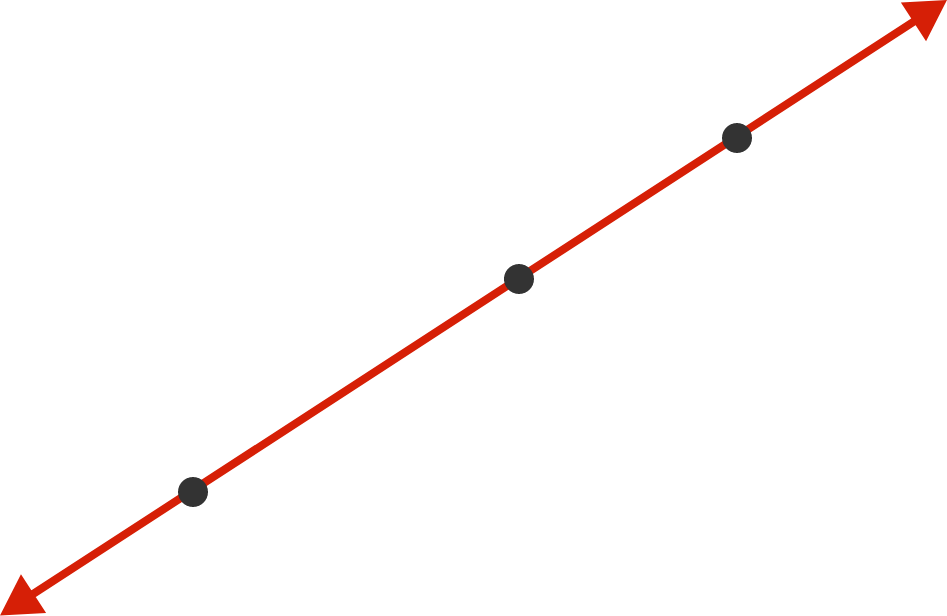If 3 points are placed on a plane such that it is not possible to draw a line through exactly two points, then the 3 points must be collinear.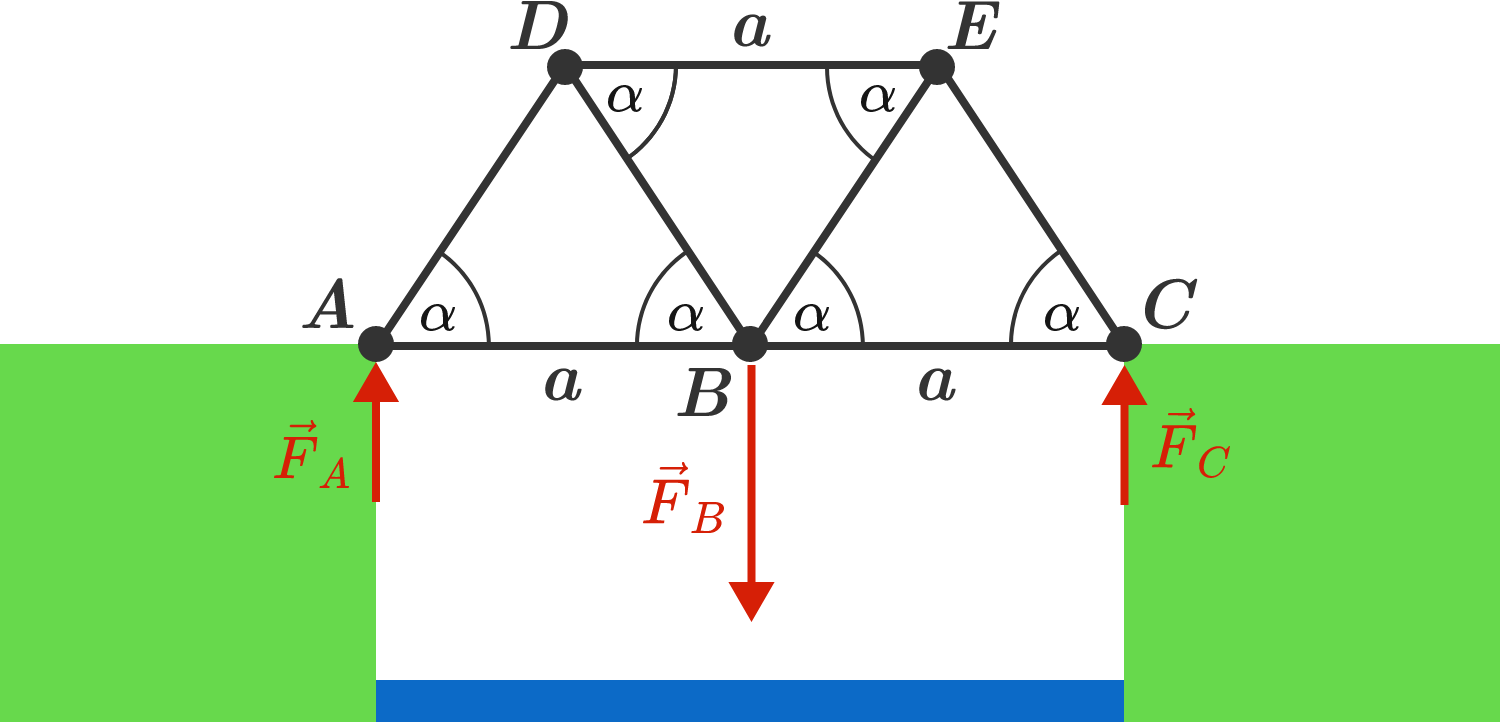A simple bridge of three triangular elements spans a river and is carried by the foundation at $A$ and $C$ in the diagram. The connecting lines between the points correspond to steel girders, each of which can bear a maximum tensile and compressive load of 10 tons.

What is the minimum angle $\alpha$ needed so that a truck with a weight of 10 tons can safely cross the bridge?

Hints:

• Assume that the weight load of the truck is concentrated at point $B$, so here a force of $\vec F_B = (10 \,\text{tons})\,\cdot \vec g$ acts on the bridge.
• The bridge is held by the opposing forces $\vec F_A = \vec F_C = - \frac{1}{2} \vec F_B$ on points $A$ and $C$. Calculate the forces acting along the steel girders.

Note: for every point of the polygon, the sum of all forces must be zero.

A function $f(n)$ is defined over positive integers as follows:

$f(n) = \begin{cases} 0 & \text{if }n\text{ is a perfect square}; \\ 1 & \text{if }n\text{ is closer to the perfect square before it than to the one after it}; \\ -1 & \text{otherwise}. \end{cases}$

For example, $f(1) = 0$ because 1 is a perfect square; $f(2) = 1$ because 2 is closer to 1 than it is to 4; $f(7) = -1$ because 7 is closer to 9 than it is to 4.

What can be said about the series $\displaystyle \sum_{n=1}^{\infty} \frac{f(n)}{n}?$

In the diagram below, two semicircles and one quarter-circle are inscribed in a unit square.

Inside one of the partitioned sections, infinitely many circles are drawn that are tangent to each other and to the boundaries. Let $r_n$ denote the radius of the $n^\text{th}$ largest circle.

Express $r_n$ in terms of $n.$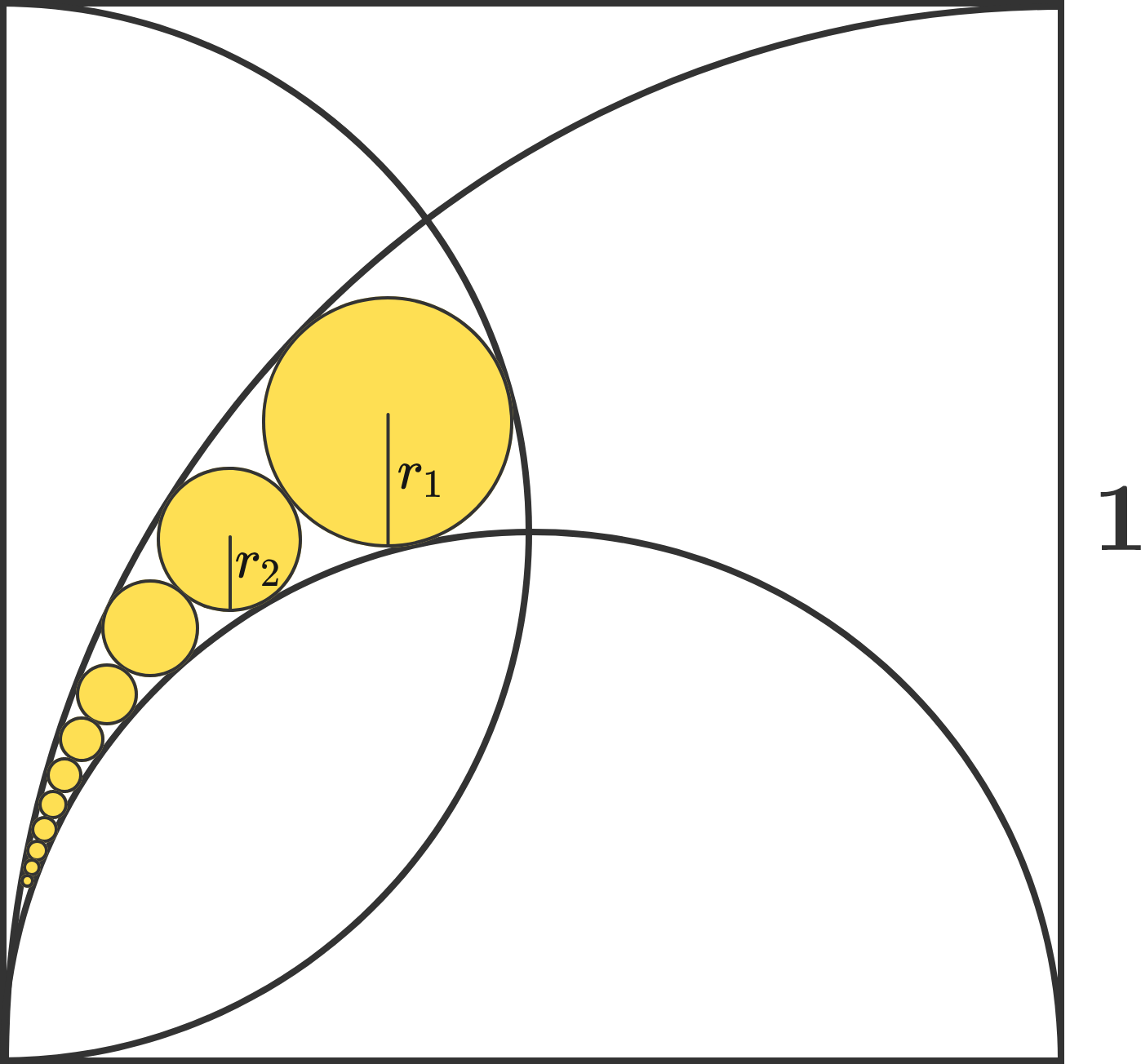Imagine a pyramid made out of blocks, where the first layer has 1 block, the second layer is a $2\times 2$ square made up of 4 blocks, the third layer is a $3\times 3$ square made up of 9 blocks, and so on, so that each $n^\text{th}$ layer is a square made from $n^2$ blocks.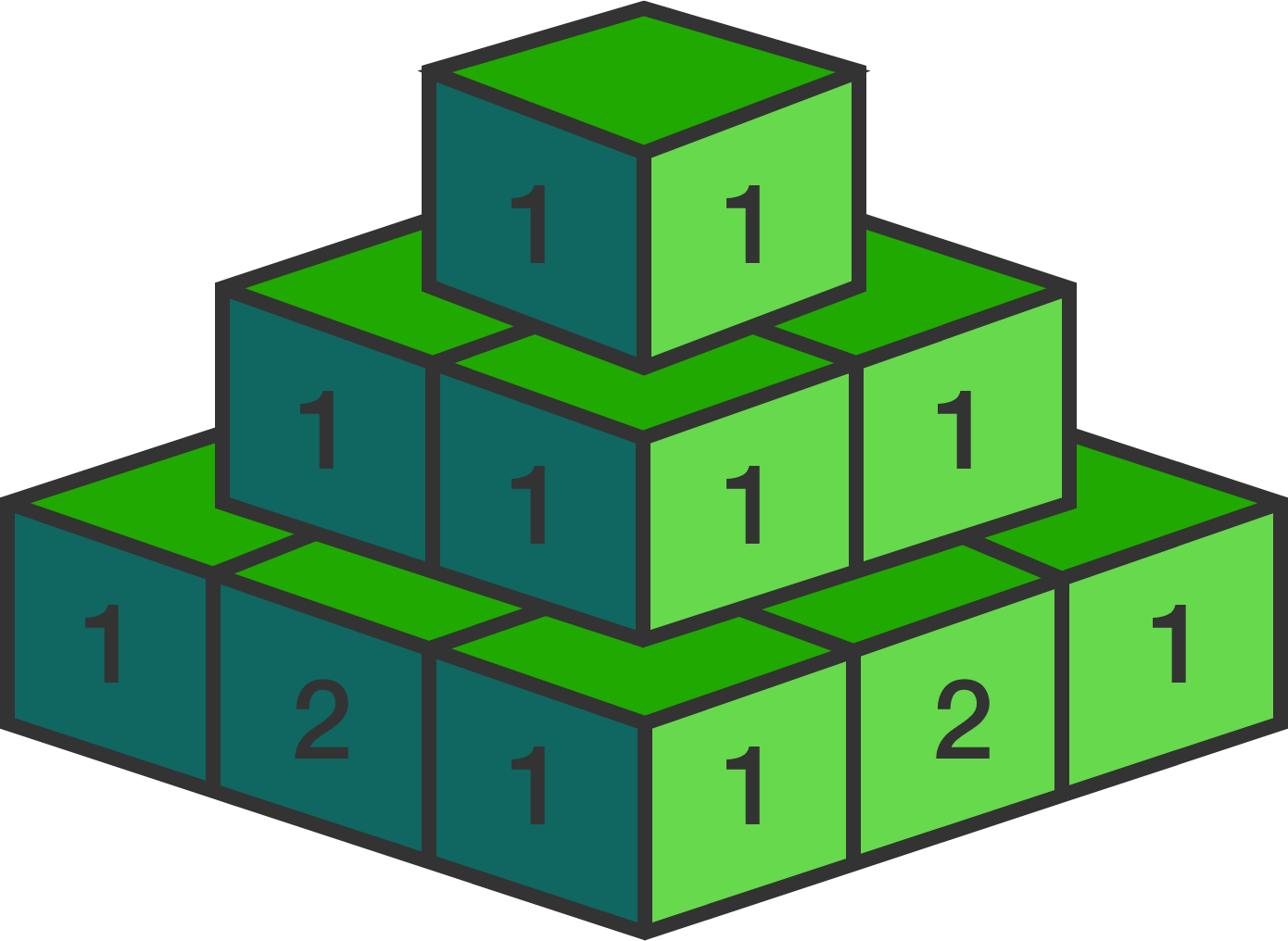Each block has a number written on it. The top block has the number 1 written on it, but every other block has the sum of all the blocks that touch its top side written on it. The first five layers are as follows: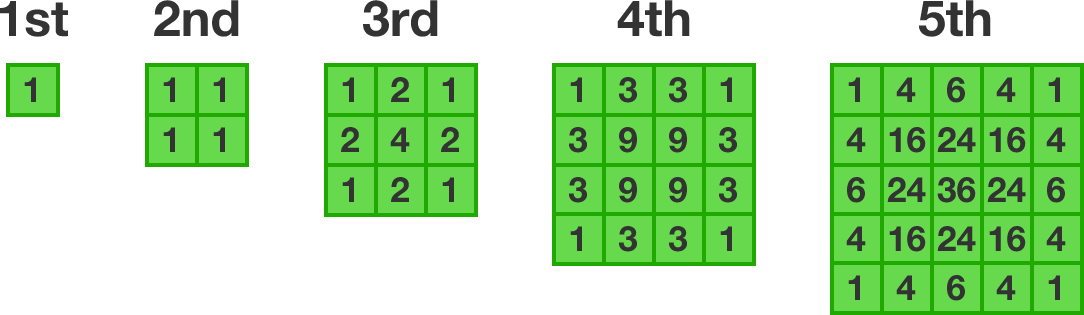What number would be written on the block that is in the $3^\text{rd}$ column and $4^\text{th}$ row of the $100^\text{th}$ layer?

Note: This problem does not require a programming solution, although you may want to use a calculator for the last step!

×